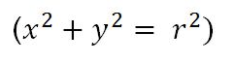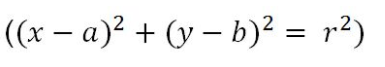The Circle Review
Full Name *
Teacher *
On a scale of 1 to 5, 1 being the best and 5 being the worst, rate yourself on how confident you are with finding the equation of a circle with centre O and radius r. *
Best
WorstOn a scale of 1 to 5, 1 being the best and 5 being the worst, rate yourself on how confident you are with finding the equation of a circle with centre (a,b) and radius r. *
Best
WorstOn a scale of 1 to 5, 1 being the best and 5 being the worst, rate yourself on how confident you are with finding the equation of a circle in general form. *
Best
WorstOn a scale of 1 to 5, 1 being the best and 5 being the worst, rate yourself on how confident you are with finding the centre and radius of a circle in any form. *
Best
Worst
On a scale of 1 to 5, 1 being the best and 5 being the worst, rate yourself on how confident you are with finding the equation of a tangent to a circle. *
Best
Worst
On a scale of 1 to 5, 1 being the best and 5 being the worst, rate yourself on how confident you are with using the discriminant to determine the number of points of contact between a line and circle. *
Best
Worst
On a scale of 1 to 5, 1 being the best and 5 being the worst, rate yourself on how confident you are with finding the point/points of intersection between a line and a circle. *
Best
Worst
Have you discussed this with your parent/carer? *